# Selection, Insertion And Bubble Sort In Python

## Introduction

In this chapter, we will discuss how to implement various sorting techniques in Python.

## SORTING

Sorting refers to arranging elements in a specific order which can either be Ascending (increasing order) or Descending (decreasing order).

For instance -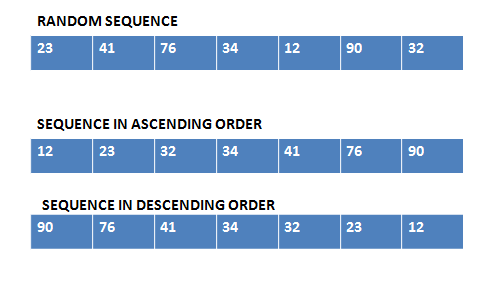There are multiple ways or techniques to sort a group of elements. Some known algorithms are,
• Bubble sort
• Insertion sort
• Selection sort
• Heap sort
• Quick sort
• Merge sort etc…
We will discuss the working and algorithm of Bubble, Selection, and Insertion sort.

## Bubble Sort in Python

Bubble sort compares two adjoining values and exchanges them if they are not in the proper order.

In this, the heaviest element or the greatest number comes at the bottom within the initial iteration.

Algorithm
1. for i in range len(L):
2. for j in range (0,len(L)-1):
3. if (L[j]>L[j+1]):
4. temp=L[j]
5. L[j]=L[j+1]
6. L[j+1]=tempWorking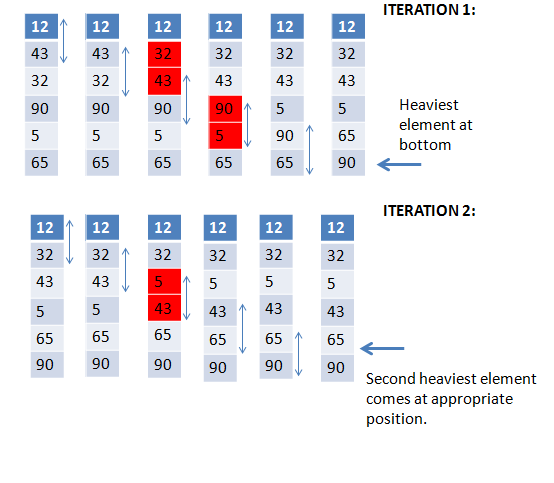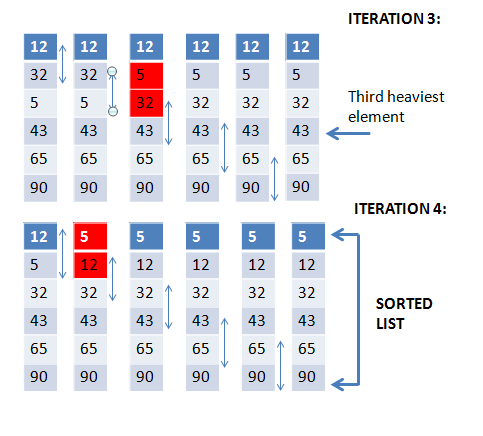## Insertion Sort in Python

Insertion sort is an algorithm that builds a sorted list, one element at a time from the unsorted list by inserting the element at its correct position in the sorted list.

It virtually divides the list into two sub-lists: sorted sub-list and unsorted sub-list.

Algorithm
1. for i in range len(L):
2. for j in range (0,i)
3. if (L[i]<L[j]):
4. temp=L[i]
5. L[i]=L[j]
6. L[j]=temp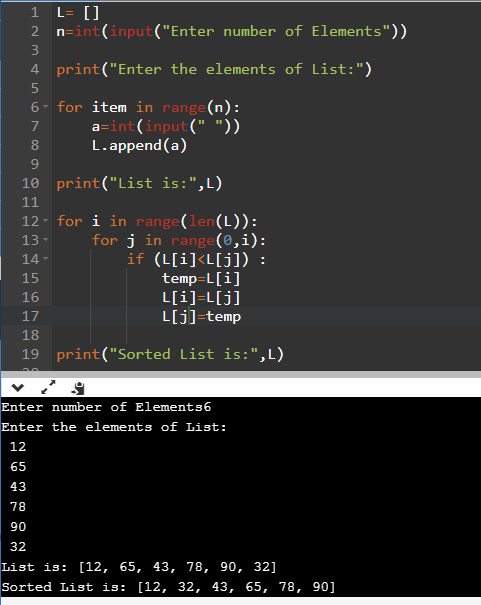Working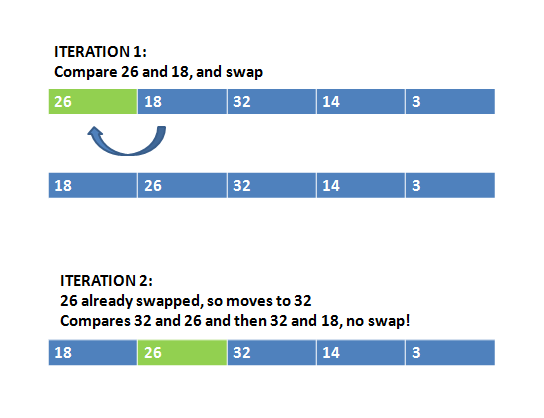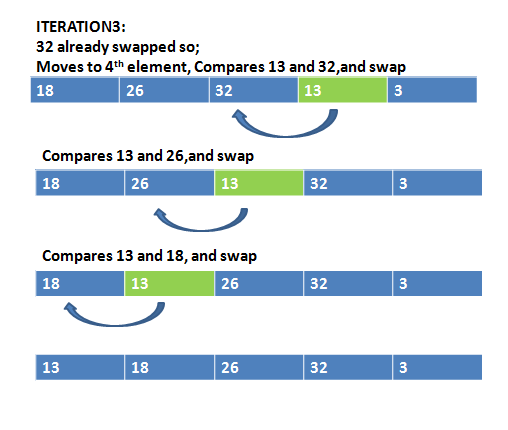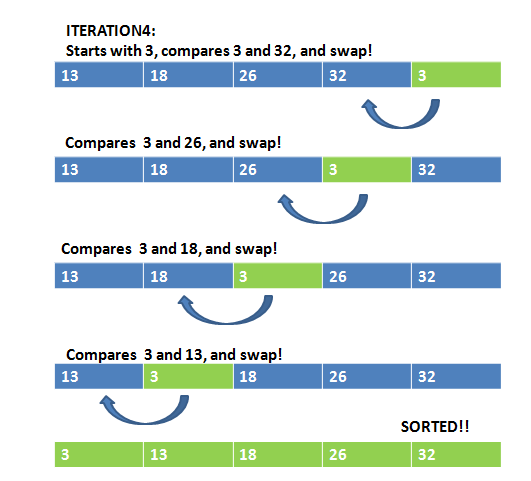## Selection Sort in Python

The basic idea of Selection sort is to repeatedly select the smallest key in the remaining unsorted list. It is also called and implemented in our systems as Exchange selection sort.

Algorithm
1. for i in range len(L):
2. for j in range (i+1,len(L))
3. if (L[i]>L[j]):
4. temp=L[i]
5. L[i]=L[j]
6. L[j]=temp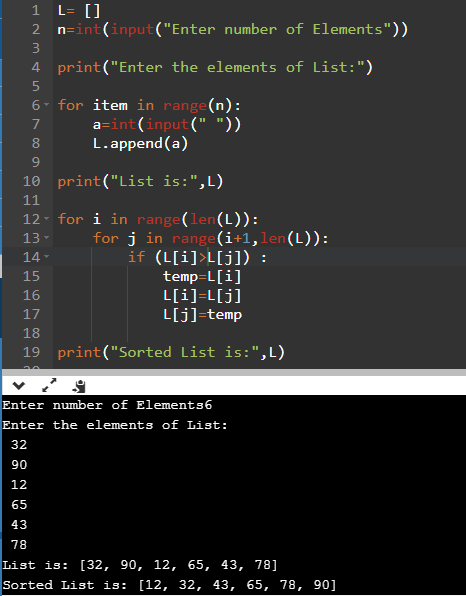Working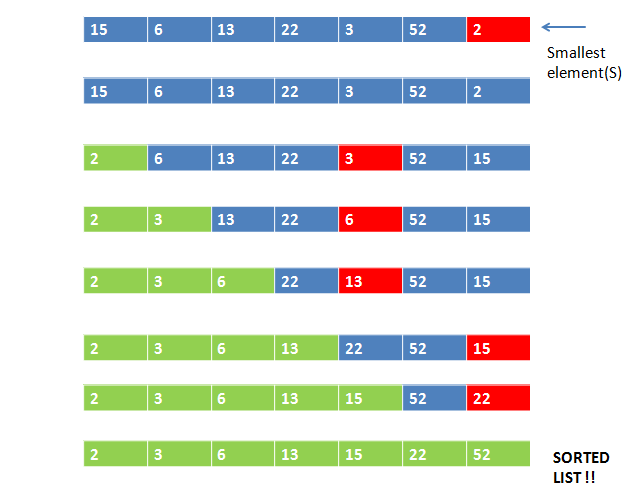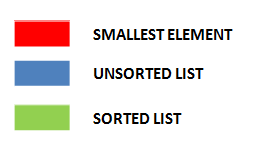## Summary

In the next chapter, we will discuss regular expressions in python.
Next » Python Regular Expression